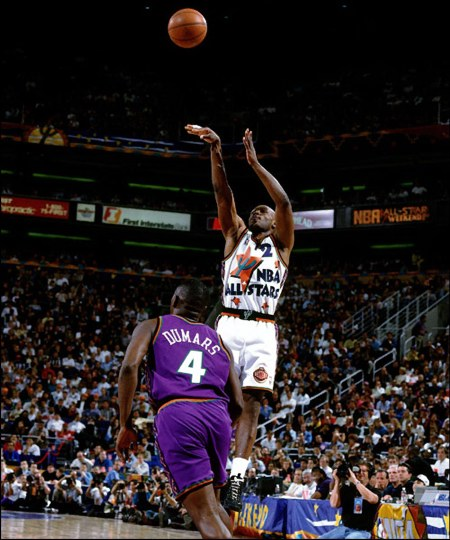Mitch RichmondAW197 / Sun conjunction

Mitchell James "Mitch" Richmond (born June 30, 1965 in Fort Lauderdale, Florida) is a retired American basketball player in the National Basketball Association. He played collegiately at Kansas State University.

http://en.wikipedia.org/wiki/Mitch_RichmondAssuming noon, 17:00 UT
Using RIYAL 3.13

Astrological Setting (Tropical - Placidus)

RIYAL Wed June 30 1965 UT 17h00m00s Lat26n07 Lon80w08 RADIX

(Moon) = 0,01 Leo                 Pluto = 14,01 Vir
Mars = 0,50 Lib                     Thereus = 14,11 Aqu r
Cyllarus = 1,33 Sag r             Echeclus = 14,24 Vir
Chariklo = 1,59 Pis r             Jupiter = 15,53 Gem
Okyrhoe = 2,30 Cap r            Chaos = 16,07 Ari
Radamantu= 2,46 Leo          Crantor = 16,33 Tau
(Ascend) = 3,04 Lib              Saturn = 17,13 Pis r
(Midheav) = 3,08 Can           Neptune = 17,27 Sco r
Pelion = 4,02 Vir                    Logos = 17,33 Can
Nessus = 6,28 Gem              CY118 = 17,48 Can
Ceto = 6,32 Tau                     Apogee = 18,04 Cap r
Pylenor = 7,28 Vir                 TD10 = 20,55 Sco r
Sun = 8,40 Can                    Pholus = 20,57 Aqu r
TL66 = 9,21 Pis r                  Chiron = 22,33 Pis r
Teharonhi= 10,10 Cap r        Amycus = 22,34 Tau
Hylonome = 11,13 Gem        Quaoar = 23,25 Lib r
Uranus = 11,30 Vir               Varuna = 24,40 Tau
Eris = 11,34 Ari                     Typhon = 25,59 Ari
Bienor = 11,51 Vir                 Elatus = 26,09 Leo
Deucalion= 12,22 Vir            Asbolus = 27,46 Can
Huya = 12,43 Leo                  Ixion = 28,14 Lib r
CZ118 = 13,20 Leo               Mercury = 28,24 Can
Node = 13,41 Gem r             Venus = 29,45 Can
Orcus = 13,52 Can                Sedna = 29,58 Ari
_____________________________________________

Focused Minor Planets

AW197 = 8Ca32
Sun = 8Ca40
OM67 = 6Cp48 r

TL66 = 9Pi21 r                 Trine

AZ84 = 9Ge06                  Semisextile

XA192 = 7Ar40                 Square
_________________

Sedna = 29Ar58
Ixion = 28Li14 r

Mercury = 28Ca24           Square
Venus = 29Ca45

RN43 = 28Sa18 r              Trine

TY364 = 29Aq14 r             Sextile
_________________

TC302 = 0Ar32 r
Mars = 0Li50

RM43 = 0Pi31 r                  Semisextile

Cyllarus = 1Sa33 r              Trine
_________________

OR10 = 15Aq05 r
Thereus = 14Aq11 r

Jupiter = 15Ge53              Trine

Pluto = 14Vi01                   Quincunx
Echeclus = 14Vi24

Chaos = 16Ar07                Sextile

MS4 = 15Sc35 r                 Square

SA278 = 14Pi32 r              Semisextile
_________________

Logos = 17Ca33
CY118 = 17Ca48

Saturn = 17Pi13 r             Grand Trine
Neptune = 17Sc27 r

TX300 = 17Aq03 r             Quincunx
OP32 = 16Sa36 r

Crantor = 16Ta33               Sextile
_________________

Eris = 11Ar34
UK126 = 12Ar49

Uranus = 11Vi30               Quincunx
Bienor = 11Vi51

Huya = 12Le43                   Grand Trine
OO67 = 12Sa16 r

Hylonome = 11Ge13           Sextile
________________________________________________

Posted to Centaurs (YahooGroups) on July 08, 2010

_____________________________________________________
_____________________________________________________